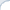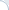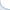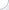I discussed my previous post with Daniel Lemire and he pointed out that integer division was also very slow. This really surprised me. What is even more surprising is that there is no vectorized integer division. Apparently, not in even in AVX2. As noted by Nathan Kurz, integer division is so bad that you can probably do better by converting integers to floating-point numbers, carrying out a vectorized floating-point division operation, and casting the result back to integer.

So, I decided to verify this hypothesis. Unfortunately, it is not possible to use single-precision floating point numbers for all possible integer values, because the significand can hold only 23 bits. This is why my first implementation uses double-precision values. Note that I implemented two versions here: one uses 128-bit vector operations (SSE4.1) and another uses 256-bit vector operations (AVX). The code is available online. The double-precision test includes functions: testDiv32Scalar, testDiv32VectorDouble, and testDiv32VectorAVXDouble. The results on (my laptop Core i7) are:

testDiv32Scalar

Milllions of 32-bit integer DIVs per sec: 322.77

testDiv32VectorDouble

Milllions of integer DIVs per sec: 466.964

testDiv32VectorAVXDouble

Milllions of integer DIVs per sec: 374.595

As you can see, there is some benefit of using SSE extensions, but not AVX. This is quite surprising as many studies found AVX to be superior. Perhaps, this is due to the fact that AVX load/stores are costly and AVX cannot outperform SSE unless the number of load/store operations is small compared to to the number of arithmetic operations.

If we don't need to deal with numbers larger than 222, single-precision format can be used. I implemented this idea and compared the solution based on division of single-precision floating-point numbers against division of 16-bit integer numbers. We are getting a three-fold improvement with SSE and only a two-fold improvement with AVX:

testDiv16Scalar

Milllions of 16-bit integer DIVs per sec: 325.443

testDiv16VectorFloat

Milllions of 16-bit integer DIVs per sec: 997.852

testDiv16VectorFloatAvx

Milllions of 16-bit integer DIVs per sec: 721.663

It is also possible to do divide integers using several CPU instructions. This approach relies on clever math, but can it be faster than a built-in CPU operation? Indeed, it can, if one computes several quotients at once using SSE/AVX instructions. This method is implemented in the Intel math library (function _mm_div_epi32) and in the Agner's library vectorclass. In the latter, all vector elements can be divided only by the same divisor. The Intel library allows you to specify a separate divisor for each vector element. On core i7, the Agner's function is only 10% faster than built-in scalar division. The Intel's function is about 1.5 times faster than scalar division. Yet, it is about 1.5 times slower than the version based on single-precision numbers.

Finally, I carried out some tets for an AMD CPU and observed higher performance gains for all the methods discussed here. In particular, the version that relies on double-precision numbers is 4 times faster than the scalar version. The Agner's vectorclass division is twice is fast as the scalar version.